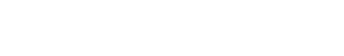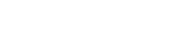Back# Top 10 Evaluation Metrics for Classification Models

October 23, 2019 Eilon Baer Predictive Models

## In a nutshell, classification algorithms take existing (labeled) datasets and use the available information to generate predictive models for use in classification of future data points. But are your models dependable? Are you tracking the right metrics for your needs? Let’s find out.

It's important to understand that none of the following evaluation metrics for classification are an absolute measure of your machine learning model’s accuracy. However, when measured in tandem with sufficient frequency, they can help monitor and assess the situation for appropriate fine-tuning and optimization.

Here are a few values that will reappear all along this blog post:

• Predicted: Outcome of the model on the validation set
• Actual: Values seen in the training set
• Positive (P): Observation is positive
• Negative (N): Observation is not positive
• True Positive (TP): Observation is positive, and is predicted correctly
• False Negative (FN): Observation is positive, but predicted wrongly
• True Negative (TN): Observation is negative, and predicted correctly
• False Positive (FP): Observation is negative, but predicted wrongly## 1. Confusion Matrix

Also known as an Error Matrix, the Confusion Matrix is a two-dimensional matrix that allows visualization of the algorithm’s performance. While this isn't an actual metric to use for evaluation, it's an important starting point.

Predictions are highlighted and divided by class (true/false), before being compared with the actual values. The matrix's size is compatible with the amount of classes in the label column. In a binary classification, the matrix will be 2X2. If there are 3 classes, the matrix will be 3X3, and so on.

This matrix essentially helps you determine if the classification model is optimized. It shows what errors are being made and helps to determine their exact type. Besides machine learning, the Confusion Matrix is also used in the fields of statistics, data mining, and artificial intelligence.

## 2. Accuracy

A classification model’s accuracy is defined as the percentage of predictions it got right. However, it's important to understand that it becomes less reliable when the probability of one outcome is significantly higher than the other one, making it less ideal as a stand-alone metric.

For example, if you have a dataset where 5% of all incoming emails are actually spam, we can adopt a less sophisticated model (predicting every email as non-spam) and get an impressive accuracy score of 95%. Unfortunately, most scenarios are significantly harder to predict.

The expression used to calculate accuracy is as follows:

Accuracy = TP + TN / TP + TN + FP + FN

## 3. Detection rate

This metric basically shows the number of correct positive class predictions made as a proportion of all of the predictions made.

Detection Rate = TP / TP + FP + FN + TN

## 4. Logarithmic loss

Also known as log loss, logarithmic loss basically functions by penalizing all false/incorrect classifications. The classifier must assign a specific probability to each class for all samples while working with this metric. The formula for calculating log loss is as follows:• Yij - Indicates if sample i belongs to class j or not
• Pij - Indicates the probability of sample i belonging to class j

In a nutshell, the range of log loss varies from 0 to infinity (∞). The closer it is to 0, the higher the prediction accuracy. Minimizing it is a top priority.

## 5. Receiver operating characteristic curve (ROC) / area under curve (AUC) score

The ROC curve is basically a graph that displays the classification model’s performance at all thresholds. As the name suggests, the AUC is the entire area below the two-dimensional area below the ROC curve. This curve basically generates two important metrics: sensitivity and specificity.## 6. Sensitivity (true positive rate)

### The true positive rate, also known as sensitivity, corresponds to the proportion of positive data points that are correctly considered as positive, with respect to all positive data points.

Sensitivity = TP / FN + TP

## 7. Specificity (false positive rate)

### False positive rate, also known as specificity, corresponds to the proportion of negative data points that are mistakenly considered as positive, with respect to all negative data points.

Specificity = FP / FP + TN

Please note that both FPR and TPR have values in the range of 0 to 1.

## 8. Precision

This metric is the number of correct positive results divided by the number of positive results predicted by the classifier.

Precision = TP / TP + FP

## 9. Recall

Recall is the number of correct positive results divided by the number of all samples that should have been identified as positive.

Recall = TP / TP + FN

## 10.  F1 score

The F1 score is basically the harmonic mean between precision and recall. It is used to measure the accuracy of tests and is a direct indication of the model’s performance. The range of the F1 score is between 0 to 1, with the goal being to get as close as possible to 1. It is calculated as per:## Watch out for overfitting

It’s important to note that having good KPIs is not the end of the story.

You will also need to keep an eye on overfitting issues, which often fly under the radar. This occurs when the model is so tightly fitted to its underlying dataset and random error inherent in that dataset (noise), that it performs poorly as a predictor for new data points.

A common way to avoid overfitting is dividing data into training and test sets. The recommended ratio is 80 percent of the data for the training set and the remaining 20 percent to the test set. You can then build the model with the training set and use the test set to evaluate the model.There is also underfitting, which happens when the model generated during the learning phase is incapable of capturing the correlations of the training set. But this phenomenon is significantly easier to detect. Your performance metrics will suffer instantly if this is taking place.

All in all, you need to track your classification models constantly to stay on top of things and make sure that you are not overfitting.